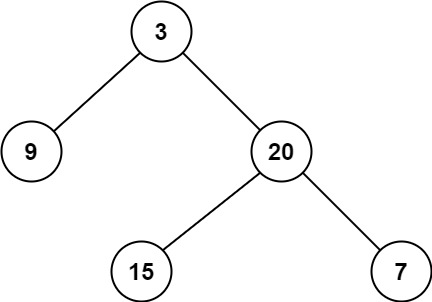## 111. Minimum Depth of Binary Tree

Given a binary tree, find its minimum depth.

The minimum depth is the number of nodes along the shortest path from the root node down to the nearest leaf node.

Note: A leaf is a node with no children.

Example 1:```Input: root = [3,9,20,null,null,15,7]
Output: 2
```

Example 2:

```Input: root = [2,null,3,null,4,null,5,null,6]
Output: 5
```

Constraints:

• The number of nodes in the tree is in the range `[0, 105]`.
• `-1000 <= Node.val <= 1000`

## Rust Solution

``````struct Solution;
use rustgym_util::*;

trait MinDepth {
fn min_depth(&self) -> usize;
}

impl MinDepth for TreeLink {
fn min_depth(&self) -> usize {
match self {
None => 0,
Some(node) => {
let node = node.borrow();
match (&node.left, &node.right) {
(None, None) => 1,
(Some(_), None) => TreeLink::min_depth(&node.left) + 1,
(None, Some(_)) => TreeLink::min_depth(&node.right) + 1,
(Some(_), Some(_)) => {
usize::min(
TreeLink::min_depth(&node.left),
TreeLink::min_depth(&node.right),
) + 1
}
}
}
}
}
}

impl Solution {
fn min_depth(root: TreeLink) -> i32 {
root.min_depth() as i32
}
}

#[test]
fn test() {
let root = tree!(3, tree!(9), tree!(20, tree!(15), tree!(7)));
assert_eq!(Solution::min_depth(root), 2);
}
``````

Having problems with this solution? Click here to submit an issue on github.